# Formulas Use Cases

Info

You can find the syntax and the role of each function and variable in the Functions and Variables Reference. See the reference to learn the use of each function and variable.

The Formulas Use Cases document collects the most common use cases of formulas encountered in every day eLegere use. You can:

• Copy & Paste the formulas and adapt them to your case.
• Use the cases as a blueprint to write your own formulas.
• Combine the cases for more complex uses.

## Index¶

Info

Each use case has the following format:

### Use Case Title

The title of the use case scenario.

#### Scenario

This paragraph explains the scenario and which necessity the formula must satisfy.

#### Where to Insert?

This paragraph explains where to insert the related formula.

#### Formula

This paragraph contains the formula and where to use it.

Each Formula section at the bottom has a summary of the functions and system variables employed in the case.

#### Commentary

This paragraph discusses how to employ the formula in similar use cases or any exception.

## Appearance¶

This section collects use cases where an application's appearance changes according to certain conditions.

### Cell Changes Color When You Record an Expense or Earnings¶

#### Scenario¶

You have a personal budgeting application recording all your expenses and earnings.

The application colors the cell "Value €" of green if the entry is an earning or red if the entry is an expense.

#### Where to Insert?¶

If you want to configure a table field's cell to change colors given certain conditions:

1. In Design Mode, navigate to Layout.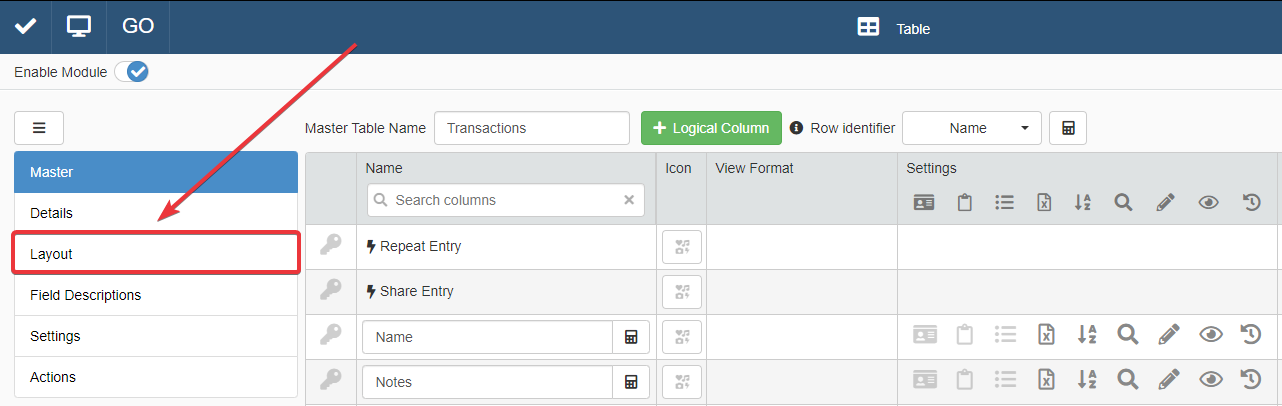2. Click on the Cell Background Color button for the cell you want to configure. The action opens the Color Settings window.3. In the Color Settings window, click on the Set Formula button to open the Formula Editor.#### Formula¶

The following formula checks if the value is positive or negative.

• If positive, the value is an earning and the cell becomes green.
• If negative, the value is an expense and the cell becomes red.

Besides earning and expenses, there could be the case where the inserted value is 0. In case the value is 0, the cell color should be gray.

 ``` 1 2 3 4 5 6 7 8 9 10 11 12 13 14 15 16 17 18 19``` ``````/* If (=IF) The amount in the field Value (\$CURRENTSTORAGE.Value) is greater (=GT) than 0, then the cell backround becomes green. Else, if (=IF) the amount in the field Value (\$CURRENTSTORAGE.Value) is lower than (=LT) 0, then the cell backround becomes red. Else, the cell backround becomes gray. */ =IF( =GT(\$CURRENTSTORAGE.Value; 0); "green"; =IF( =LT(\$CURRENTSTORAGE.Value; 0); "red"; "gray" ) ) ``````

Question

How do you specify a particular color in a formula? You must insert between quotation marks (`""`) a code specifying the color. eLegere supports any standard HTML color format.

Employed Functions and System Variables

#### Commentary¶

You can employ the same formula pattern also for other Layout components in Design Mode.For example, if you want to change the cell's text color according to its value, insert the formula by clicking on the Cell Text Color button.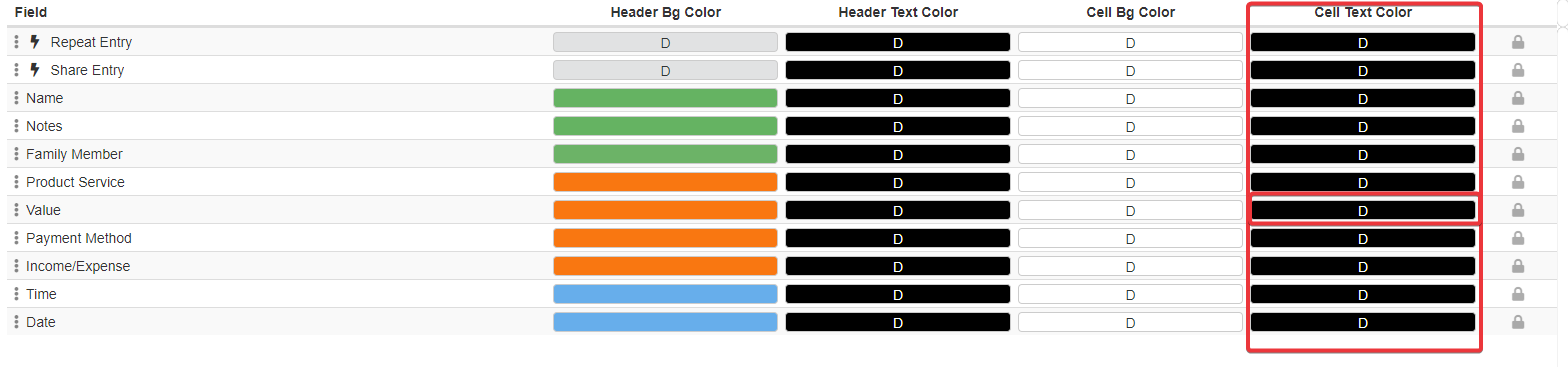You can use as many Comparison formulas as you want to add conditions and the related colors. In case you want to add more conditions to be satisfied, add an `=AND()` or `=OR()` as first parameter of the `=IF()`. Then add more Comparison functions (e.g. `=GT()`, `=LT()`, `=GTE()`, `=LTE()`, `=EQ()`).

Use more than two nested `=IF()` functions inside one each other to add more conditions to the condition's tree.

Example

An example of nested conditions in a formula.

 ``` 1 2 3 4 5 6 7 8 9 10 11 12 13 14 15 16``` ``````=IF( [Insert a function to check a value here]; "Insert the color code or name here"; =IF( ...; =IF( ...; =IF( ...; =IF( ... ) ) ) ) ) ``````

### Change Row Color When the Status Changes¶

#### Scenario¶

You have an application that manages the reimbursements.

A Lookup drop-down list records the status of the request (e.g. Draft, Submitted, Approved, Rejected, Revised).

The application must highlight with a different color the entry according to the Status. For example:

• Draft is yellow.
• Revised and Submitted are white.
• Approved is green.
• Rejected is red.

#### Where to Insert?¶

If you want to configure a row to change colors given certain criteria:

1. In Design Mode, navigate to Layout.2. Turn on the Toggle Advanced Settings toggle switch. This action opens the Advanced Settings.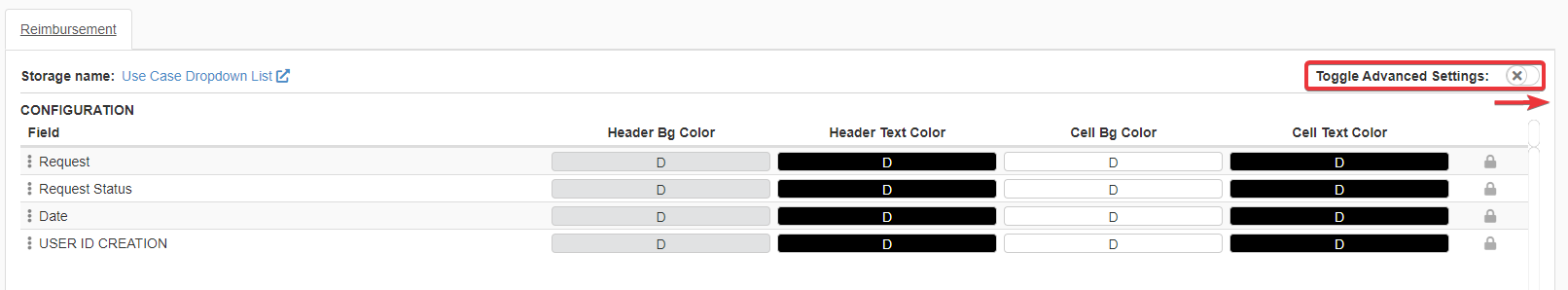3. Click on the Background button (D) below the Row Colors. The action opens the Row background color window.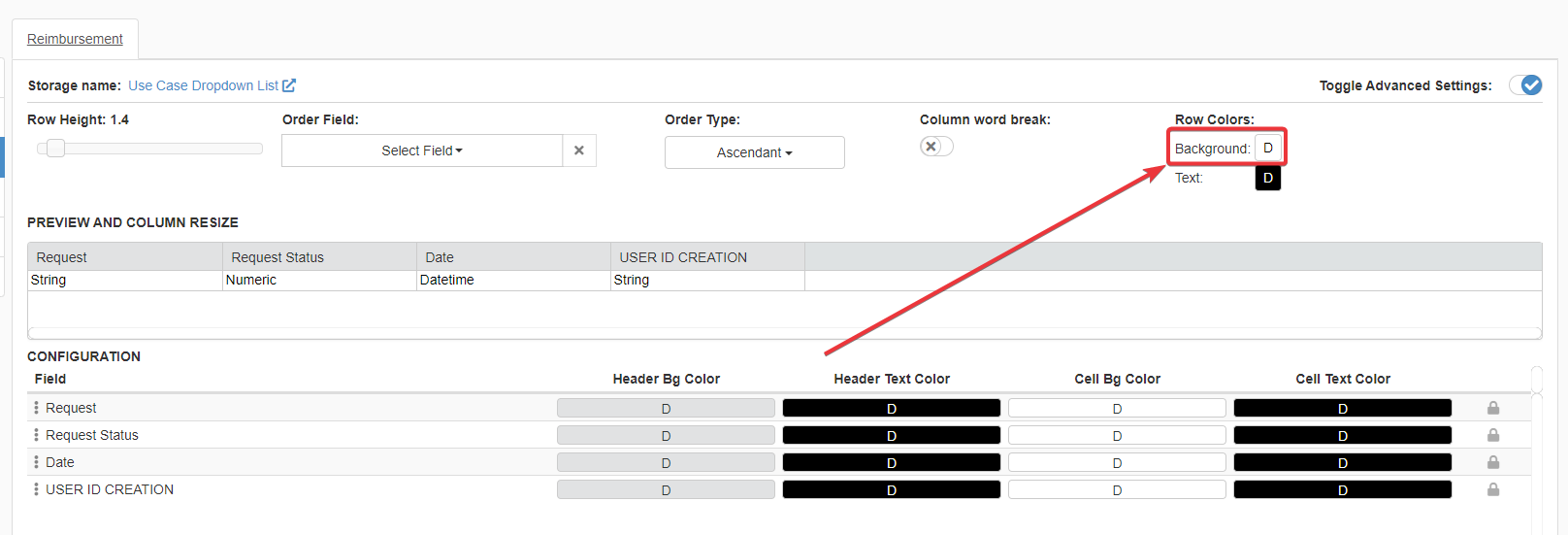4. In the Color Settings window, click on the Set Formula button to open the Formula Editor.#### Formula¶

The formula checks the recorded option in the Lookup drop-down list field Status. Each recorded option has an Id from 1 to 5.

Depending on the value of Status, the formula specifies a color for that status. The application displays then the row using that color.

 ``` 1 2 3 4 5 6 7 8 9 10 11 12 13 14 15 16 17 18 19 20 21 22 23 24 25``` ``````/* Based on (=SWITCH)      the value of field Status (\$CURRENTSTORAGE.Status):      - in case (=CASE) it is 1 (eg: Draft), the cell must be yellow.      - in cases (=CASES) 2 and 3 (e.g Revised and Submitted), white.      - in case (=CASE) 4 (Approved), green.      - in case (=CASE) 5 (Rejected), red.      - in all other cases (=DEFAULT) gray. Note that - You need at least one =CASE() or =CASES() inside =SWITCH(). - You can list as many =CASE() and =CASES() as you want. - =DEFAULT() is optional. If you don't specify =DEFAULT(), the formula won't color the row if the value is not listed in one of the =CASE() or =CASES(). */ =SWITCH(     \$CURRENTSTORAGE.Status;     =CASE(1; "yellow");     =CASES(=LIST(2; 3); "white");     =CASE(4; "green");     =CASE(5; "red");     =DEFAULT("gray") ) ``````

Question

How do you specify a particular color in a formula? You must insert between quotation marks (`""`) a code specifying the color. eLegere supports any standard HTML color format.

Employed Functions and System Variables

#### Commentary¶

You can employ the same formula pattern also for other Layout components in Design Mode.For example, if you want to change the cell's text color according to the value: insert the Formula by clicking on the Cell Text Color button.If you need a specific color in case the value doesn't satisfy any of the `=CASE()` conditions: add `=DEFAULT()` as last parameter of the `=SWITCH()` function. `=DEFAULT()` has only one parameter specifying the default value if no `=CASE()` conditions are satisfied.

Example

 ```1 2 3 4 5 6``` ``````=SWITCH( \$CURRENTSTORAGE.fieldValue; =CASE(...; ...); ... =DEFAULT([Insert here the default value that you want.]) ) ``````

## Calculation¶

This section collects use cases where cell fields calculate their values automatically.

### Display the Number of Completed Task in a Daily Task Manager¶

#### Scenario¶

The application is a Task Management application where:

• Each row is a day.
• The Detail contains a row for each task of the day.

For each day, the application must display the number of completed task out of the total number of tasks for that day.

#### Where to Insert?¶

If the Designer wants a field that updates in real time, you need to insert the formula in a Logical Column. Logical Columns are not fields existing on the Storage but entities that calculate in real time the required data.

1. In Design Mode, click on the Add Logical Column button on the Master panel.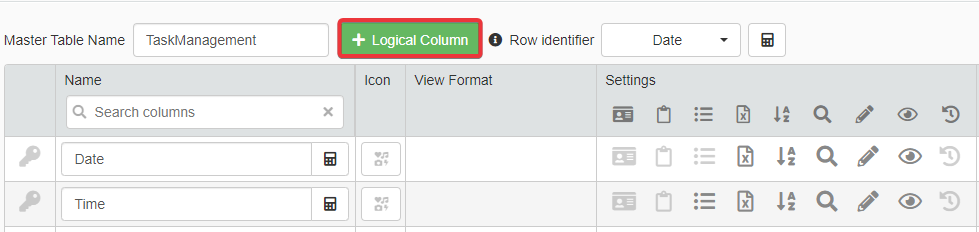2. Choose Calculation type for the Logical Column and where to insert the Logical Column in the table.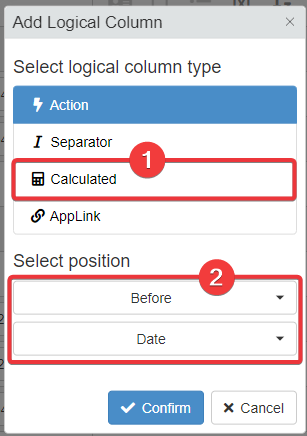3. The actions in steps 1-2 move the user to the Logical sub-panel. Add a name to the new Logical Column (e.g. Tasks Progressions).4. Move to the Master table's Formula panel. Click on the icon of the Logical Column to open the Formula Editor.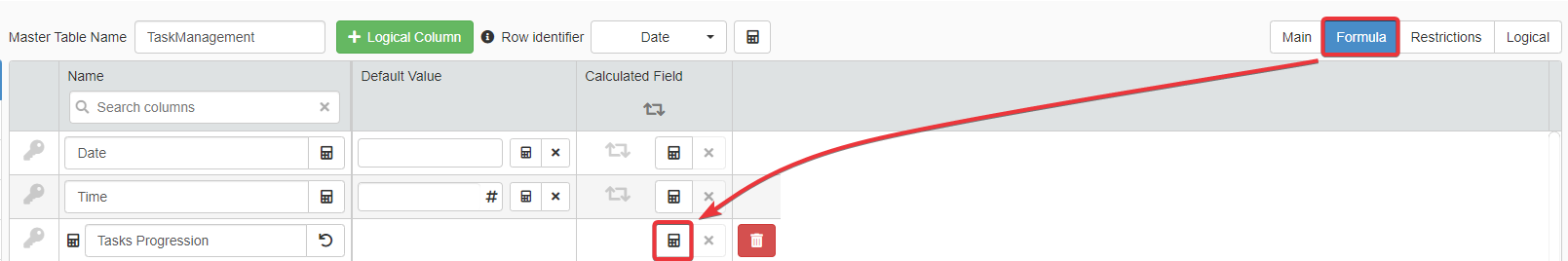#### Formula¶

The Task Management application has a Detail containing a series of tasks for each day. The Detail is called dailyTasks. Each row in dailyTask has a flag to sign each task as Completed (i.e. the Completed field).

Each Master row should show Logical Column with the total number of tasks and the number of completed task.

Insert the formula in a Logical Column's Formula Editor.

Question

In this case, the value must be a text (String) instead of a number (Numeric). This because Numeric field values don't allow inserting alphanumeric characters or strings like `\`, `@`, `task`, and so on.

 ``` 1 2 3 4 5 6 7 8 9 10 11 12 13 14 15 16 17 18 19 20 21 22 23 24 25 26 27 28 29 30 31 32``` ``````/* The formula creates the text "X out of Y" joining (=CONCAT) the calculated values below: - (1) The number of completed tasks in the Detail (=AGGREGATEDETAILS, "COUNT"), - (2) The text " out of " (a simple text between ""), - (3) The total number of tasks in the Detail (=AGGREGATEDETAILS, "COUNT"). (1) You obtain the count of completed tasks by: querying (=AGGREGATEDETAILS) the dailyTasks Detail (\$CURRENTSTORAGE.\$DETAILS.\$dailyTasks.Id_Task) asking to count ("COUNT") the rows considering only (=EQ) the rows which column Completed (#dailyTasks.Completed) is flagged (\$TRUE) Note: The condition as third parameter in the =AGGREGATEDETAILS() must reference the column field to be checked through the Detail's Storage Name (#dailyTasks). (2) Note the spaces at the beginning and the end. =CONCAT() joins everything together without spaces, unless you insert the spaces. (3) You need only the total number. =AGGREGATEDETAILS() and "COUNT" without condition is enough. */ =CONCAT( =AGGREGATEDETAILS( \$CURRENTSTORAGE.\$DETAILS.\$dailyTasks.Id_Task; "COUNT"; =EQ(#dailyTasks.Completed;\$TRUE) ); " out of "; =AGGREGATEDETAILS( \$CURRENTSTORAGE.\$DETAILS.\$dailyTasks.Id_Task; "COUNT" ) ) ``````
Employed Functions and System Variables

#### Commentary¶

If you want to calculate data on the fly that you don't need to record, use a Calculated Logical Column. Calculated Logical Columns calculates any formula and displays their result in real time.

If you have to create a text using a mix of field cells' values and fixed text, use the `=CONCAT()` function. `=CONCAT()` supports as many String type parameters as the Designer wants.

Example

You could use the `=CONCAT()` to create a unique code starting from other fields. You use functions like `=RIGHT()`, `=LEFT()`, and `=SUBSTRING()` to take strings and compose them through `=CONCAT()`.

The `=AGGREGATEDETAILS()` function has multiple employments; counting the number of rows in a Detail is one of them. You can use `=AGGREGATEDETAILS()` to sum, find the highest or lowest value or obtain the numeric average value of a field on the Detail.

### Calculating an Item Price including the VAT (%)¶

#### Scenario¶

The application should calculate the final cost of the item taxes (VAT) included.

The user chooses a country from a Lookup drop-down list. The item's final price should change according to the country's local VAT.

#### Where to Insert?¶

In the Formula sub-panel, insert the formula under the Calculated Field column in correspondence to the field's row.

1. In the Design Mode, go to the table containing the price with VAT's Calculated field.

2. Click on the Formula sub-panel.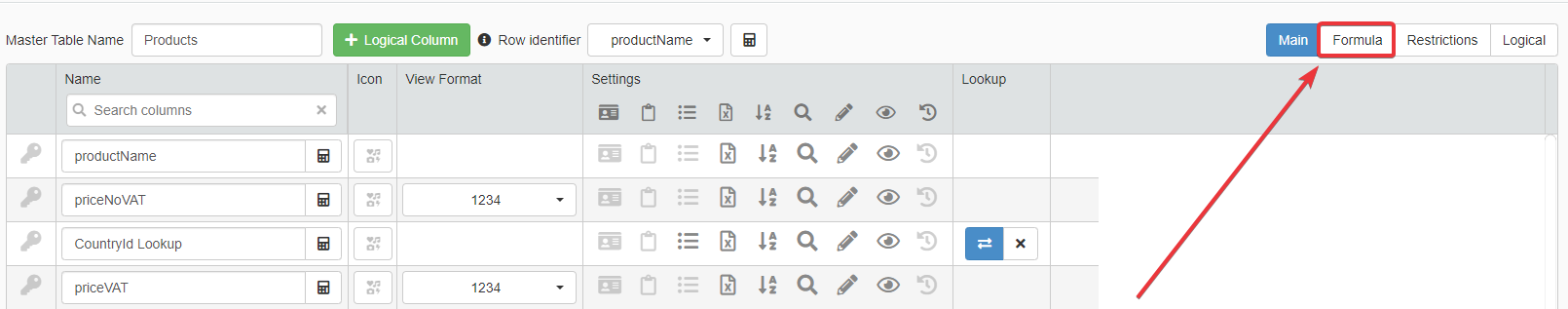3. Click on the icon under the Calculated Field column corresponding to the price with VAT field.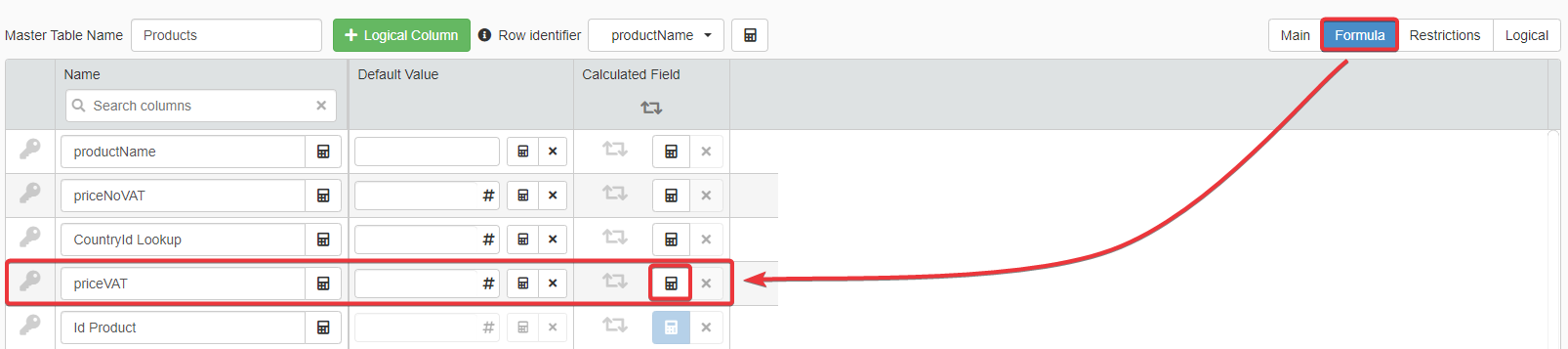#### Formula¶

The formula makes two arithmetic calculations by picking up the VAT from the countries' Storage (called in the example `sCountriesInfo`).

Note

Applications can represent a percentage as a integer number (es. 100%, 50%). In Storages, Numeric type fields records the percentages (%) as decimal numbers. E.g. 22 % = 0.22, 7% = 0.07

The field CountryId_Lookup records the Countries' reference (Id) from `#sCountriesInfo`. `#sCountriesInfo` contains the country's name and its VAT (%).

Insert the formula in the field that will record the final price VAT included.

 ``` 1 2 3 4 5 6 7 8 9 10 11 12 13 14 15 16 17 18 19 20 21 22 23 24 25 26 27``` ``````/* To obtain the final prices, the formula takes the item or service price without VAT (\$CURRENTSTORAGE.priceNoVAT) and adds (+) the price without VAT multipled (*) for the chosen country VAT percentage (%) (=LOOKUP). (The VAT % is a decimal number like 0.22, 0.10) Note that in the current Storage you don't have the chosen country's VAT. You have to retrieve it through the Id of the chosen country (\$CURRENSTORAGE.CountryId_Lookup): Using =LOOKUP: from the table sCountriesInfo (#sCountriesInfo) You identify the column with the information you need, i.e. the VAT. (#sCountriesInfo.VAT) You compare (=EQ) the value of the IdCountry field (#sCountriesInfo.IdCountry) The chosen country option's Id (\$CURRENSTORAGE.CountryId_Lookup). If the two values are equal, this is the Country you need and =LOOKUP() retrieves the VAT for the calculation. */ \$CURRENTSTORAGE.priceNoVAT + (\$CURRENTSTORAGE.priceNoVAT * =LOOKUP(      #sCountriesInfo;      #sCountriesInfo.VAT;      =EQ(            #sCountriesInfo.IdCountry;            \$CURRENSTORAGE.CountryId_Lookup      ) )) ``````

Note

`#sCountriesInfo.VAT` stores the VAT (%) as decimal number; so, multiplying the price without VAT (i.e. `priceNoVAT`) for the `#sCountriesInfo.VAT`'s value is enough to calculate the Valued-added tax.

Employed Functions and System Variables

#### Commentary¶

You can use the same formula pattern for each case where you must retrieve a value from another Storage and use it for calculations.

Use the `=LOOKUP()` function and the third parameter to identify the value you are looking for.

Example

You can use the same use case for differentiated VAT percentages according to a product or service category. (E.g. 4% for food, 15% for books, 10% electricity and gas.)

### Record the Current Date When You Add a New Entry¶

#### Scenario¶

In an application, creating a new row should fill a Date o Date-Time field with the current date automatically.

#### Where to Insert?¶

The current date should be a Default Value: every time the user inserts a new row in the application, the field must have that particular value. In this case, the value of the current Date or Date and Time (if the field is Date-Time).

1. In the Design Mode, go to the table containing the Date or Date-Time field.

2. Click on the Formula tab.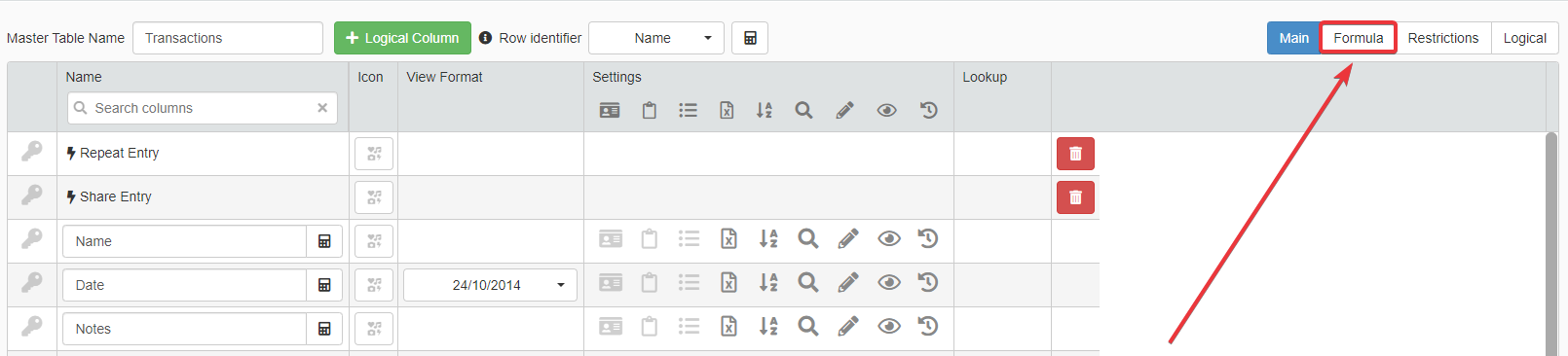3. Next to the desired Date or Date-Time field, click on the icon in the Default Value column.#### Formula¶

The formula is just a variable pointing to the current Date or Date-Time (depending on the field's type).

 ```1 2 3``` ``````// Retrieve the current date and time (\$CURRENTDATE) \$CURRENDATE ``````
Employed Functions and System Variables

#### Commentary¶

You can use the `\$CURRENTDATE` system variable within String type fields to point to the current date.

Use `=CONCAT()` functions if you want to add a Date to a text.

Example

The formula below creates a sentence starting from a String type field (i.e. Username) and the `\$CURRENTDATE` variable.

 `1` ``````=CONCAT(\$CURRENTSTORAGE.Username; " has registered the request in the following date: "; =FORMATDATE(\$CURRENTDATE; "dd/mm/yyyy")) ``````
Note that you need the `=FORMATDATE()` inside the `=CONCAT()` function. `\$CURRENTDATE` represents the current Date in the eLegere proprietary format. You need `=FORMATDATE()` to format the Date in a readable format; e.g. `dd/mm/yyyy` or `mm-dd-yyyy`.

You can find here a list of format options for the `=FORMATDATE()`'s third parameter here.

This section collects use cases where you configure notifications' content and behavior through formulas.

### Sending a Notification When a Request is Submitted¶

#### Scenario¶

An application enables the employees to request holidays and time-off.

The application must warn through an email the Supervisors of any submitted request waiting approval or rejection.

In eLegere, Designers can configure applications to send email notification automatically. The Designers to do so must create a Send Email type Custom Action. Designers can define receivers and content dynamically using formulas.

#### Where to Insert?¶

You must create a Send Email Custom Action first. Use the formulas to define the notification's receivers, Subject, and Body dynamically.

Follow the steps below to create the Send Email Custom Action:

1. In the Design Mode, click on the icon on the top-right corner of the interface.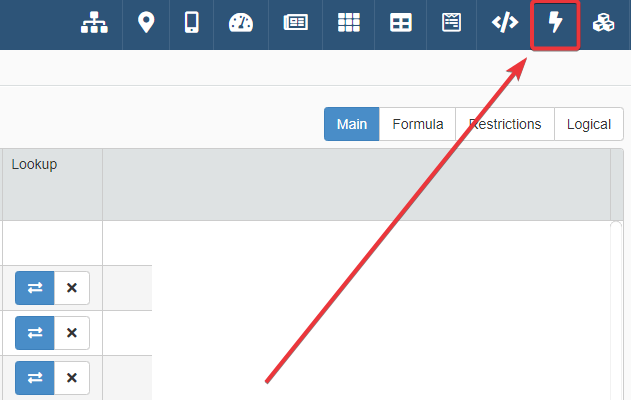2. Click on the New Action drop-down list and choose Send Email as option.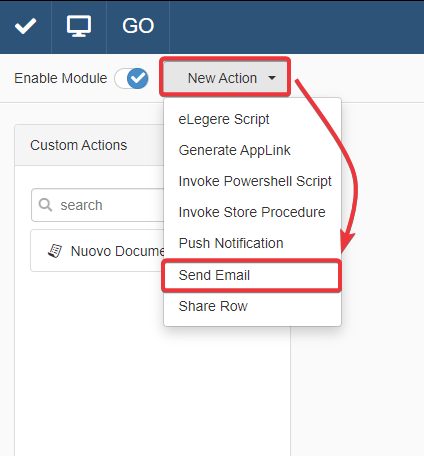3. In the left side-bar with the Custom Actions' list, click on the action you have created.4. Disable the Manual toggle switch. The action runs when an event triggers the activation.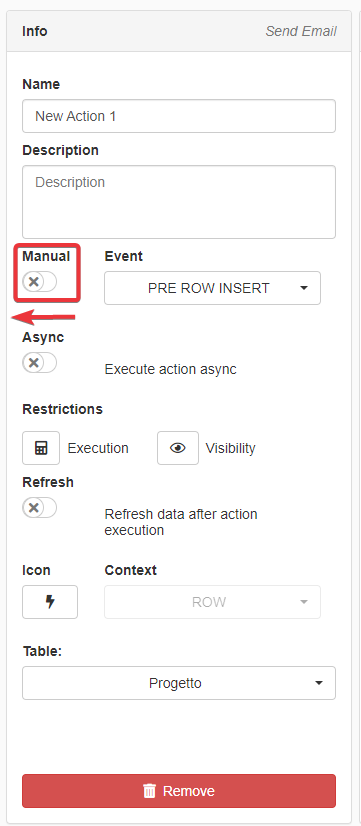5. Choose POST ROW INSERT as option from the Event drop-down list. This option triggers the action at each new entry on the table.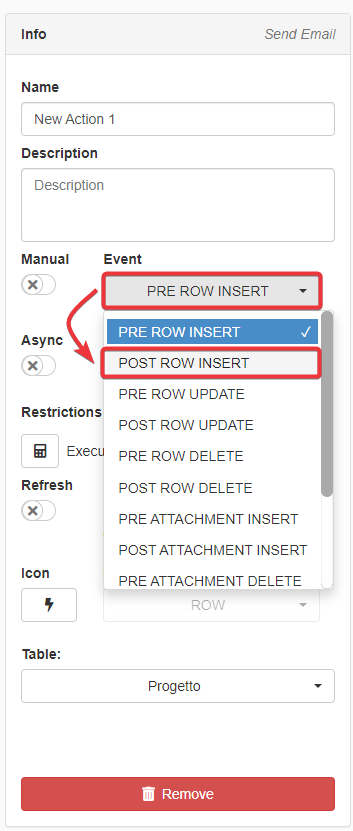Designers can insert formulas:

1. In the To: field through the icon.
2. In the Subject.
3. In the Body.Note

Inserting formulas either in the Subject or the Body requires enclosing the formula between two squared brackets `[]`. Inside Subject and Body in Send Email Custom Actions, applications interpret formulas outside squared brackets `[]` as standard text and won't run them.

#### Formula¶

The Custom Action Send Email employs three separated formulas in the three sections To:, Subject, and the Body.

You must insert the formulas in these sections of the Send Email Custom Action sub-panel.

1. For the To:, you need a formula to retrieve the supervisors' list.
2. For the Subject, you need a formula to record the request's Date and Time.
3. For the Body, you need a link to the request to speed up the approval o rejection. It is optional but useful.Employed Functions and System Variables
##### To: Field¶

For the To:, you need a formula to retrieve the supervisors' list.

Click on the icon next to the To: field.

Note

In this case, you don't need enclosing the formula between two squared brackets `[]`. You are inserting the formula in the Formula Editor.

The function `=GROUPUSERSEMAILS()` specifies in the To: to take all the email addresses of the "Supervisors" User Group's members.

The Custom Action will send the email to everyone belonging to the "Supervisors" User Group.

 `1` ``````=GROUPUSERSEMAILS("Supervisors") ``````
##### Subject¶

For the Subject, you need a formula to record the request's Date and Time.

Note

The formula goes into a Subject field. It requires the squared brackets to be interpreted as formula (`[]`).

Type a standard template text for the email's Subject. For example:

 `1` `````` A request needs approval ``````

Add the formula to insert the Date at the end of the standard template text.

The formula's task is taking the current Date (`\$CURRENTDATE`) and displaying it (`=FORMATDATE`) according to the format "day-month-year hour:minutes" (`"dd-mm-yy hh:mm"`).

`\$CURRENTDATE` retrieves the current Date and Time.

The function `=FORMATDATE()` formats the current Date and Time according to the following format: "dd-mm-yy hh:mm".

 `1` ``````[=FORMATDATE(\$CURRENTDATE; "dd-mm-yy hh:mm")] ``````
##### Body¶

For the Body, you could insert a link to the request to speed up the approval o rejection. It is optional but useful.

Note

The formula goes into the Body text. It requires the squared brackets to be interpreted as formula (`[]`).

Write a template text for the Body. For example:

 `1` `````` A employee has submitted a new request waiting for approval. Click on the link to view the request. ``````

The formula goes into a Body's text.

Creates a link (`=GENERATEROWLINK`) to the specified row in the application's Storage (`#sHolidayRequests`).

The formula yields a link redirecting to the application and the new entry. It points to the application and Storage `#sHolidayRequests` that contain the requests.

 `1` ``````[=GENERATEROWLINK(#sHolidayRequests)] ``````

#### Commentary¶

You can use the same formulas even for notification about rows' changes, deletion or attachments (upload, download or deletion). Choose a different trigger from the Event drop-down list from the Info panel.

Example

• POST ROW UPDATE Choose from the Event drop-down list if you want to warn the User Group after any edits.
• POST ROW DELETE Choose from the Event drop-down list if you want to warn the User Group after any deletion.
• POST ATTACHMENTS INSERT Choose from the Event drop-down list if you want to warn the User Group after any attachments' uploads.
• POST ATTACHMENTS DELETE Choose from the Event drop-down list if you want to warn the User Group after any attachments' deletion.

You can find all the supported Date/Time formats for the third parameter of `=FORMATDATE()` function here.

If you want to insert a link to a different application using `=GENERATEROWLINK()`:

1. Insert as first parameter the Storage name of the other application.
2. Insert as second parameter the field to consider from the Storage.
3. Insert as third parameter the field designated as Primary Key in the application.
4. The Id of the external application between quotation marks (`""`).

Example

An example of use to link a row to an application different from the current one.

 `1` ``````[=GENERATEROWLINK(#sHolidayRequests; #sHolidayRequests.IdEntry; \$CURRENTSTORAGE.IdEntry; "b87e99b5-8757-4443-b61b-ad04de55bb32")] ``````

## Restriction¶

This section collects use cases where you can restrict the displayed application's content or operations (e.g. adding, editing, or deleting rows).

### Disable Changes When the Project Status is Completed¶

#### Scenario¶

A Project Management application must block any change to a project's row when its status is Completed.

Only members of the "Chief Directors" User Group can modify any project at any stage, included Completed.

#### Where to Insert?¶

If you want to add any restriction to a table regarding adding, editing, cloning or deleting rows:

1. In Design Mode, navigate to the Settings sub-panel.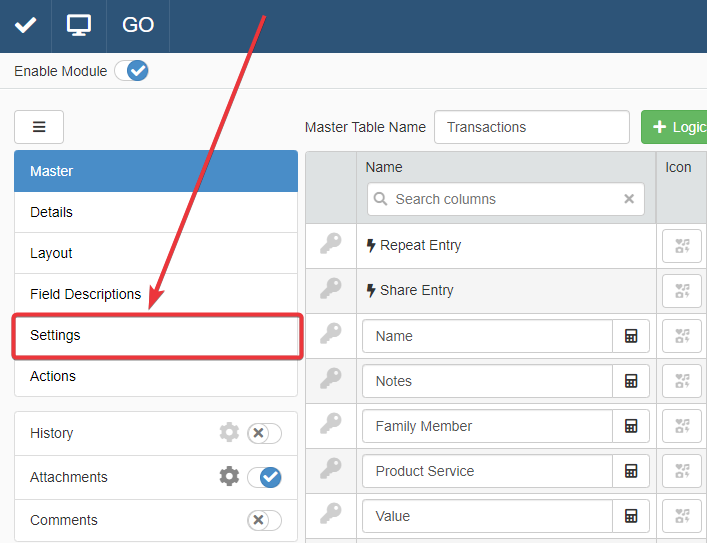2. The Settings sub-panel has a tab for each application's table. Navigate the desired table (1) to apply the restriction.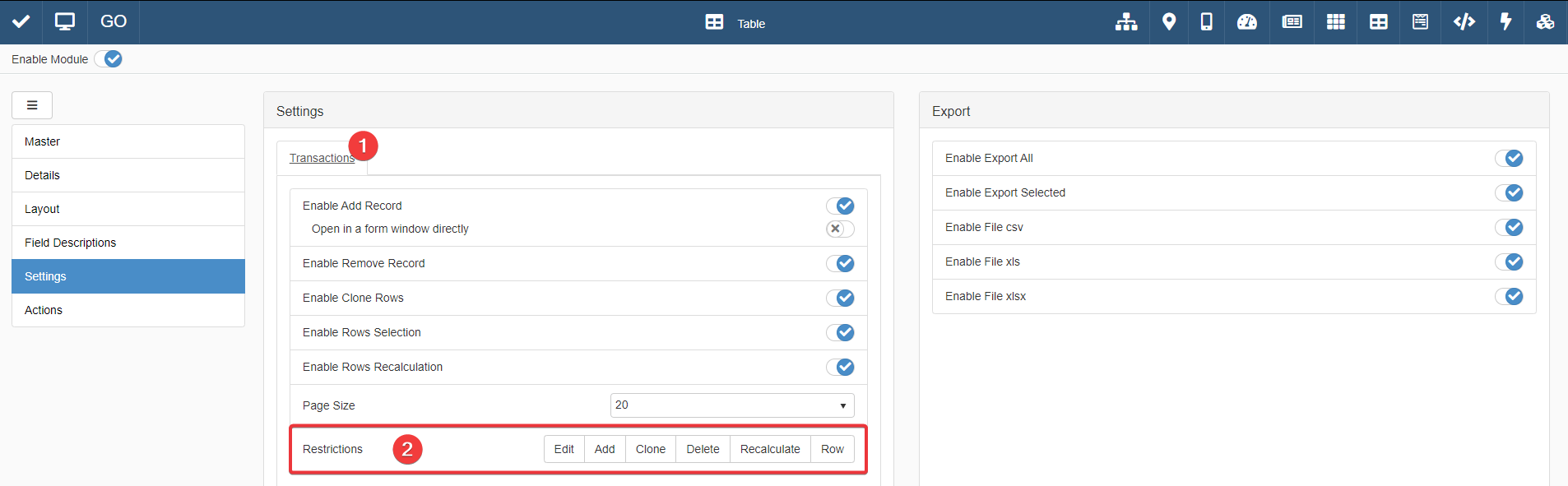3. According to the kind of restriction, choose either Edit, Add, Clone, Delete, Recalculate, and Row in the Restriction (2) section of the Settings.

In this case scenario, you should apply the formula restriction to Edit.

#### Formula¶

Insert the formula in Settings > [Table's Tab] > Restrictions > Edit.

The formula displays either all the rows or certain rows according to the User's Group.

 ``` 1 2 3 4 5 6 7 8 9 10 11 12 13``` ``````/* Row is editable when at least one of the listed conditions is satisfied. or (=OR): - The current user (\$CURRENTUSER.GROUPNAMES) belongs to the "Chief Directors" User Group (=CONTAINS). - The project status is not (=NEQ) Completed (5). */ =OR( =CONTAINS(\$CURRENTUSER.GROUPNAMES; "Chief Directors"); =NEQ(\$CURRENTSTORAGE.projectStatus; 5) ) ``````
Employed Functions and System Variables

#### Commentary¶

You can create more complex conditions for restriction by using Comparison functions like `=AND()`, `=GT()`, `=GTE()`, `=LT()`, =`LTE()`, `=NEQ()` or `=EQ()`.

### Make Mandatory a Motivation Field If the Supervisor Rejects a Request¶

#### Scenario¶

An application enables the employees to request holidays and time-off.

If a Supervisor rejects a submitted request, the Supervisor must fill the field Motivation with a motivation.

#### Where to Insert?¶

If you want to add any restriction to specific fields' cells, you must go to the Restrictions sub-panel.

1. Navigate to the table containing the field.

2. Click on the Restriction sub-panel button.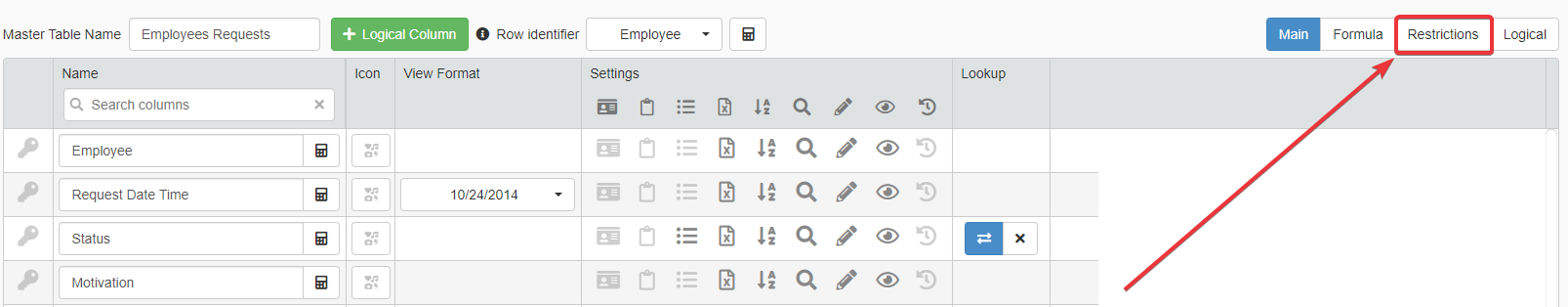3. Click on the Cell Mandatory Restriction button next to the field you want to restrict.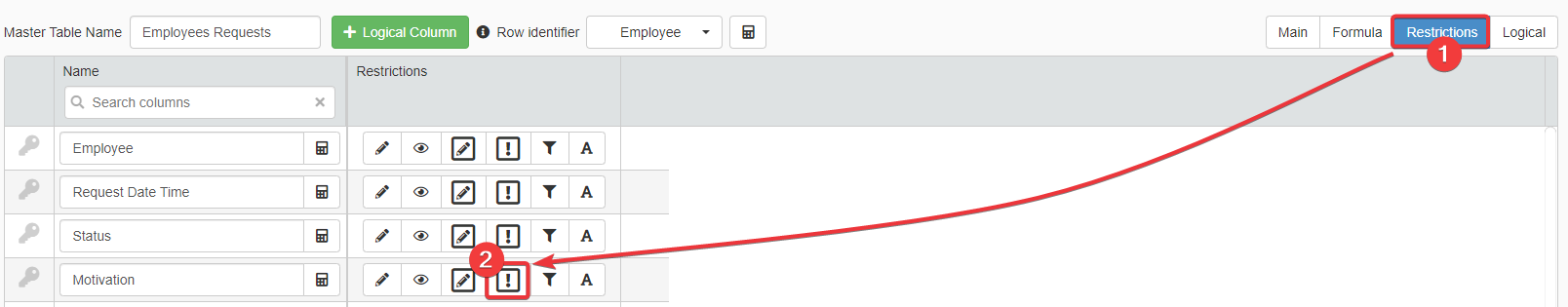Insert the formula in the Cell Mandatory Restriction for the Motivation field.

#### Formula¶

The field Motivation records a text explaining the status' choice motivation.

If the status is Rejected, then the user must insert a motivation for the rejection.

 ```1 2 3 4 5 6``` ``````/* The field Motivation becomes mandatory when the request status (\$CURRENSTORAGE.requestStatus) is (=EQ) Rejected (3). */ =EQ(\$CURRENSTORAGE.requestStatus; 3) ``````
Employed Functions and System Variables

#### Commentary¶

You can add any Comparison function in a Cell Mandatory Restriction to set conditions that make a field mandatory if verified. For example: `=NEQ()`, `=ISNULL()`, `=ISNOTNULL()`, `=GT()`, `=GTE()`, `=LT()`, and `=LTE()`.

### Employees Can Only Add New Rows While Managers Can Update or Delete Entries¶

#### Scenario¶

In a budgeting application, each user can record the expenses made, but they cannot make any change nor delete their entries.

In this scenario, only the budget's managers can update or delete the rows in the application.

#### Where to Insert?¶

If you want to add any restriction to a table regarding adding, editing, cloning or deleting rows:

1. In Design Mode, navigate to the Settings sub-panel.2. The Settings sub-panel has a tab for each application's table. Navigate the desired table (1) to apply the restriction.3. According to the kind of restriction, choose either Edit, Add, Clone, Delete, Recalculate, and Row in the Restriction (2) section of the Settings.

In this case scenario, you should apply the formula restriction to Edit and Delete.

#### Formula¶

The following formula verifies that the user belongs to the "Managers" group.

 ``` 1 2 3 4 5 6 7 8 9 10``` ``````/* The formula checks if the current user's User Groups list (\$CURRENTUSER.GROUPNAMES) includes (=CONTAINS) "Managers". \$CURRENTUSER is a system variable that refers to the active user's information. \$CURRENTUSER.GROUPNAMES retrieves the current user's User Groups list. =CONTAINS() checks if "Managers" is in the user's User Groups list. */ =CONTAINS(\$CURRENTUSER.GROUPNAMES; "Managers") ``````
Employed Functions and System Variables

#### Commentary¶

In the example above, only users belonging to the "Manager" User Group can either edit or delete any row of the table.

If you want to enable more than one User Group for editing or deleting rows: you can use the function `=CONTAINSLIST()` and `=LIST()` as second parameter. `=CONTAINSLIST()` works like the `=CONTAINS()` function, but `=CONTAINSLIST()` checks if a list contains one or more elements from `=LIST()`.

Example

The formula checks if the user belongs to either "Managers" or "Product Owners" or "Developers" User Groups. If the user belongs to at least one of the User Groups, the application enables both editing and deleting.

 `1` ``````=CONTAINSLIST(\$CURRENTUSER.GROUPNAMES; =LIST("Managers"; "Product Owners"; "Developers")) ``````

The case scenario takes as example a restriction for editing and deleting. If you want to restrict Add, Clone, and Recalculate to certain User Groups, the same formula works as well for these actions.

### The Users Should View Only the Rows They Have Created¶

#### Scenario¶

In an application managing the family budget, each user must view only their own rows with their own expenses or income. In this way, their privacy is kept.

#### Where to Insert?¶

If you want to add restrictions about what rows a user can see in an application:

1. In Design Mode, navigate to the Settings sub-panel.2. The Settings sub-panel has a tab for each application's table. Navigate the desired table (1) to apply the restriction.3. According to the kind of restriction, choose either Edit, Add, Clone, Delete, Recalculate, and Row in the Restriction (2) section of the Settings.

In this case scenario, you should apply the formula restriction to Row.

#### Formula¶

The formula displays to the current user only the rows they have created.

Question

USER_ID_CREATION field is a System Field: each Storage you create has this field. Refer to USER_ID_CREATION in your formulas if you want to enable actions or display only the rows that the current user created.

 ``` 1 2 3 4 5 6 7 8 9 10 11 12 13``` ``````/* The formula checks if the row's creator (\$CURRENTSTORAGE.USER_ID_CREATION) is (=EQ) the current logged user (\$CURRENTUSER.LOGONNAME). Note: USER_ID_CREATION is a system field created for each Storage. USER_ID_CREATION records who has created the row in the application. The formula below verifies that the creator recorded in USER_ID_CREATION corresponds to the current user. If they are the same, the row appears to the current user. */ =EQ(\$CURRENTSTORAGE.USER_ID_CREATION; \$CURRENTUSER.LOGONNAME) ``````
Employed Functions and System Variables

#### Commentary¶

Every time you want to restrict the displayed rows, you can use any Comparison function. For example: `=NEQ()`, `=CONTAINS()` or `=CONTAINSLIST()`.

The pattern is comparing a one or more values with other values: if the values are the same, the row satisfies the condition and the application displays it.

### Employees Should View Only Their Rows and the Managers All the Rows¶

#### Scenario¶

A task management application records what each user has done and when.

Employees and Managers view different sets of rows.

User Group Row
Employees Only the rows they have created.
Managers All the rows of all the users.

Each row has a field recording who has performed the task.

#### Where to Insert?¶

If you want to add restrictions about what rows a user can see in an application:

1. In Design Mode, navigate to the Settings sub-panel.2. The Settings sub-panel has a tab for each application's table. Navigate the desired table (1) to apply the restriction.3. According to the kind of restriction, choose either Edit, Add, Clone, Delete, Recalculate, and Row in the Restriction (2) section of the Settings.

In this case scenario, you should apply the formula restriction to Row.

#### Formula¶

Each row has a Lookup drop-down list field CompanyMembersId_Lookup to assign the user to a specific tasks. CompanyMembersId_Lookup refers to the list of company members (`#CompanyMembers`). The Storage `#CompanyMembers` has among its fields the Email field. The formula uses this field on `#CompanyMembers` to identify the rows of users that aren't in the User Group "Managers".

The formula checks if the user is a Manager or Employees. The formula grants either total access (Manager) or display only certain rows according to the User Group (Employees).

 ``` 1 2 3 4 5 6 7 8 9 10 11 12 13 14 15 16 17 18 19 20 21 22 23 24``` ``````/* OR (=OR) The current user belongs to the "Managers" User Group and view everything (=CONTAINS) OR the current user belongs to Employees Group (=CONTAINS) and (=AND) must view only the rows with their name (=EQ). The application identifies from the list of employees the user through their email address (=LOOKUP) If someone accesses the application and doesn't belong to any User Group, they will view an empty Table. */ =OR( =CONTAINS(\$CURRENTUSER.GROUPNAMES; "Managers"); =AND( =CONTAINS(\$CURRENTUSER.GROUPNAMES; "Employees"); =EQ( \$CURRENTSTORAGE.CompanyMembersId_Lookup; =LOOKUP( #CompanyMembers; #CompanyMembers.IdCompanyMembers; =EQ(#CompanyMembers.Email; \$CURRENTUSER.EMAIL) ) ) ) ) ``````
Employed Functions and System Variables

#### Commentary¶

You can use the formula pattern above to add as many restrictions and conditions as you want.

Add to the `=OR()` parameters as many User Groups as you want through the `=CONTAINS()` function. If a User Group can see only the rows satisfying certain condition, enclose them in the `=AND()` function together with the `=CONTAINS()` function.

Use the function `=LOOKUP()` to retrieve any information outside the current application's Storage.

Example

An example of multiple `=AND()` function specifying multiple cases in the `=OR()`.

 ``` 1 2 3 4 5 6 7 8 9 10 11 12 13 14``` ``````=OR( =CONTAINS(\$CURRENTUSER.GROUPNAMES; "Insert here User Group Name"); ... =AND( =CONTAINS(\$CURRENTUSER.GROUPNAMES; "Insert here User Group Name"); [Formula with condition] ); =AND( =CONTAINS(\$CURRENTUSER.GROUPNAMES; "Insert here User Group Name"); [Formula with condition]; ...; [Formula with condition] ) ) ``````

### Restrict Products from a Drop-Down List according to the Selected Category¶

#### Scenario¶

An application manages and tracks orders from a warehouse.

The user should choose an item category and be able to view and choose only the products of that category.

#### Where to Insert?¶

productCategory is a Lookup drop-down list field. Users choose the Product category from this drop-down list.

productList is a Multiselection field recording one or more products from the Storage `#Products`.

You must insert the formula as a Restriction Formula for the Multiselection.

1. In the Design Mode, go to the table containing the two fields.

2. Click on the Create the Lookup Relation button () of the Lookup field you want to apply the restriction formula.3. In the options window, click on the icon corresponding to the Restriction Formula.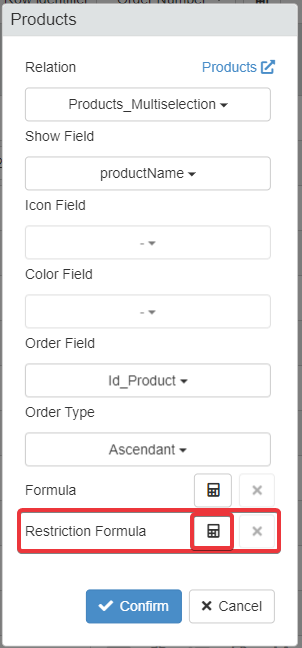#### Formula¶

The formula shows in the product's list only the items belonging to the chosen category.

 ``` 1 2 3 4 5 6 7 8 9 10 11 12 13 14 15 16 17 18``` ``````/* The formula displays only the items (=LOOKUPLIST) corresponding (=EQ) to the category chosen in the drop-down list field productCategory. You don't have on the current storage the list of items. You must retrieve the list from the Storage #Products. The =LOOKUPLIST() function retrieves for a list only the items satisfying a condition. The user will view only this set of elements. =EQ() verifies that the two parameters are equal. =LOOKUPLIST must display only the items belonging to the same category chosen in the drop-down list productCategory. */ =LOOKUPLIST( #Products; #Products.Id_Product; =EQ(#Products.productCategory; \$CURRENTSTORAGE.productCategory) ) ``````
Employed Functions and System Variables

#### Commentary¶

Every time you have two Lookup drop-down lists or one Lookup drop-down list and a Multiselection field, use the `=LOOKUPLIST()` function to filter the items according to value of one of the two fields.

As condition, you can use any function that compares two values; for example: `=EQ()`, `=NEQ()`, `=GT()`, `=LT()`, `=GTE()`, and `=LTE()`. You can join as many functions as you like through the `=AND()` and `=OR()` functions.

### Allow Choosing Certain Project Statuses according to the Current Status¶

#### Scenario¶

You have an application to record the status of different projects.

Once the project owner has chosen a new status for the project, they shouldn't revert it to previous stages.

#### Where to Insert?¶

You must insert the formula as a Restriction Formula in the drop-down list Lookup options' window.

1. In the Design Mode, go to the table containing the Lookup drop-down list.

2. Click on the Create the Lookup Relation button () of the Lookup field you want to apply the restriction formula.3. In the options window, click on the icon corresponding to the Restriction Formula.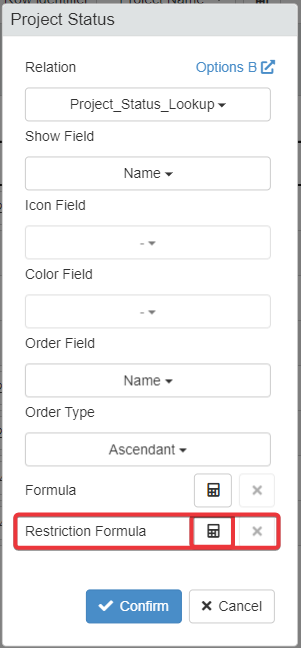#### Formula¶

The Lookup drop-down list is projectStatus field and takes the options from the Storage sProjectStatuses.

sProjectStatuses contains the following projects' statuses with their related Id:

Id Status
1 Draft
2 Submitted
3 Approved
4 Rejected

The default value is the Id 1 (i.e. the Draft status) in Table > Formula > Default Value for the field.Insert the formula in the Lookup drop-down list's Restriction Formula.

 ``` 1 2 3 4 5 6 7 8 9 10 11 12 13 14 15 16 17 18 19 20 21 22 23``` ``````/* if (=IF) The project status is (=EQ) DRAFT , then show the options DRAFT and SUBMITTED (=LIST). Else, if (=IF) the status project is SUBMITTED, then show the options SUBMITTED, APPROVED, and REJECTED (=LIST). Else, display the options APPROVED and REJECTED (=LIST). =IF() executes an action or provides a value according to the value of the first parameter. =EQ() compares one field value with another value. In this case, the actual project status with the Id of DRAFT 1. =LIST() supports the manual insertion of options and values. */ =IF( =EQ(\$CURRENTSTORAGE.projectStatus; 1); =LIST(1;2); =IF( =EQ(\$CURRENTSTORAGE.projectStatus; 2); =LIST(2;3;4); =LIST(3;4) ) ) ``````

Example

`=LIST(1;2)` means that the formula must display from the Storage sProjectStatuses only the options with Id 1 and 2.

Employed Functions and System Variables

#### Commentary¶

You can set up as many conditions as you want to display certain values from the options' Storage in a Lookup drop-down list.

As condition, you can use any function that compares two values; for example: `=EQ()`, `=NEQ()`, `=GT()`, `=LT()`, `=GTE()`, and `=LTE()`. You can join as many functions as you like through the `=AND()` and `=OR()` functions.

You can also employs the `=CONTAINS()` function and the `\$CURRENTUSER.GROUPNAMES` system variable to show certain sets of options only to specific User Groups.

Example

An example showing how to differentiate options in a Lookup or Multiselection field by the User Group.

 ```1 2 3 4 5 6 7 8 9``` ``````=IF( =CONTAINS(\$CURRENTUSER.GROUPNAMES; "Project Manager"); =LIST(1;2); =IF( =CONTAINS(\$CURRENTUSER.GROUPNAMES; "Task Owners"); =LIST(2;3;4); =LIST(3;4) ) ) ``````